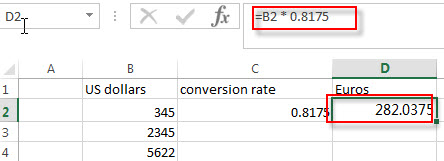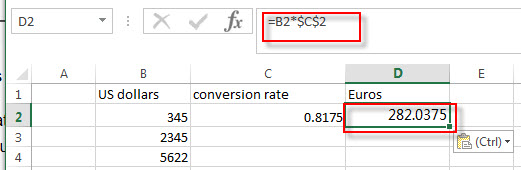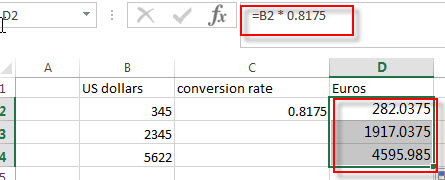# How to convert Currencies in Excel

This post will guide you how to convert from a given currency to other specific currencies, such as, how to convert the USD currency to EUR currency. How to convert one currency value to another currency’s value with excel formula.

## Convert Currency

If you want to convert one currency to another, you can write an excel formula to apply the conversion rate to existing data. Such as, to convert currency from US Dollars to your local currency (such as: EUR), you should know the conversion rate firstly, then the US dollar value multiplied by the conversion rate to get the value of your local currency.

For example, the conversion rate for US dollars to euros is 0.8175, it means that 1 USD dollar is equal to 0.8175 Eruos. So we can write down the following formula to convert currency from US dollars to Euros.

`=B2 * 0.8175`Of you can enter the conversion rate into one cell, such as: C2, then you can use the following formula:

`=B2*\$C\$2`Then you can drag the AutoFill Handle down to other cells to apply the same formula to convert currency for all cells in range B2:B4.